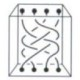## Ethiopian Multiplication

byOne of our recent (oh my goodness has it really been seven years???) grads just sent me this Youtube video of Ethiopian Multiplication, with a note that this reminded her of History of Mathematics.  Which, of course, made me totally happy.

This method of multiplication is also called Egyptian Multiplication (because it was done in Egypt) and Russian Peasant Multiplication (although the Peasant part might be intended as a bit of a pejorative).

Here’s the basic idea:  Suppose you want to multiply two numbers like 14 and 12.  You could use your fingers, of course, but here’s another way:

Start with the two numbers on top.  Halve one, ignoring any remainders or fractions, and double the other, stopping when you get to 1.

14 & 12

7 & 24

3 & 48  [See how I ignored the fact that halving 7 leaves 1 left over?]

1 & 96  <— Stop here.

Now look at the numbers on the right.  Some are across from an even number: in this case, 12 is across from the original 14.  Ignore those, and add the rest.  So we’ll add 24, 48, and 96, which were across from odd numbers, and get 168.  And that’s the product!  Isn’t that cool?

(I think it would be fantastic to write a book called 25 ways to multiply.  I only have about 13 at the moment, though.)

Here’s the video!

Number photo from gokuro.

### 16 Responses to “Ethiopian Multiplication”

1.Learner Says:

Amazing & Interesting

2.Jason Dyer Says:

Could you list them (the 13 ways)?

3.Ξ Says:

Jason, I started to reply and then realized I could just make that a blog post and ask other people to add different versions. So I’ll do that. 🙂

4.Chris Says:

This method can be approached recursively. Each step, except the final one, is identical to the previous.

(define (mult a b)
(if (= a 1) b
(+ (if (odd a) b 0) (mult (/ a 2) (* b 2)))))

The integer division and multiplication can be replaced by single bitwise right and left shifts. (I hope this pseudo-code displays ok.)

5.Barry Leiba Says:

You’re actually doing it in binary, generating (2^(N-1))*B on the right at the Nth stage. Then by taking the ones where the left side is odd, you’re taking the ones where the corresponding position in the binary representation of A is 1: reading from bottom up, you have 1 1 1 0, the binary representation of 14.

So there’s no surprise at all that this works… it’s basically the way a digital computer does it.

6.Rick Regan Says:

Just another take on the binary angle…

14 is 1110 in binary and 12 is 1100. Multiply the two in binary: 1100 x 1110 (think of 1110 as being on the bottom — I can’t draw it that way since the formatting won’t work). The partial products, in order, are 0000 (0), 11000 (24), 110000 (48), 1100000 (96). The nonzero products are copies of the top number, 12, shifted left — doubled — an appropriate number of times. Adding the partial products gives 10101000 (168).

7.Ξ Says:

I really like all the different ways that people look at this problem and see why it works. I usually justify this method by nothing that if you double one number and halve the other exactly, then the product is the same. So 14×12 is the the same as (14/2)x(12×2). The problem comes when you ignore a remainder.

14×12 is the same as 7×24, and that would be the same as 3.5×48. But we’re only left with 3×48. That means that the new product will be “off” by 0.5×48, which is 24 — not coincidentally, the number, above the 48. So whatever is across from 1 at the end is most of the product, and you have to add on the missing pieces (like that 24), which are always across from odd numbers since that’s when you run into trouble from dividing.

(And really, this is the same as the binary explanations without using the word binary.)

8.Rick Says:

Here’s another viewpoint without the word “binary”: 12*14 = 12*(8 + 4 + 2) = 96 + 48 + 24 = 168

9.Alexander Bogomolny Says:

Here’s a Java applet implementation:

http://www.cut-the-knot.org/Curriculum/Algebra/PeasantMultiplication.shtml

10.Steve Says:

“I think it would be fantastic to write a book called 25 ways to multiply”

Shouldn’t that be 5×5 ways to multiply?

11.shuka Says:

Very interesting, I am an Ethiopian but I don’t know this method.

12.jack Says:

It’s an interesting method this one, but it doesn’t work too fast.
You have to remember all the “doubles” along the way…

Compared with 14 x 12 = 14 x 10 + 14 x 2, for example…

13.Ξ Says:

Steve, you’re absolutely right! That would be the right name.

shuka — I know that there are examples of this from Egypt, but I don’t actually know enough about the documentation for this being Ethiopian.

And jack, my own view is that I’m undecided on this. I don’t think remembering doubles is inherently any harder than remembering what 4×2 is, say, although certainly I could do 14×12 in my head and that part would be harder with doubles. (Although I’d probably do it on my fingers, doing 100+60+8).

14.BOBBY Says:

•Ξ Says: